# Heights and Distance Questions and Answers

## Heights and Distance Questions

In this page we will recap through some basic information related to Heights and Distance and then we will be solving Heights and Distance Questions and Answers. Heights and Distance is an important topic for examination point of view. The topic comprises questions where height of a building, angle of elevation, and depression are given for another building, which is close by.### The angle of Elevation

It can be better understood by considering a situation where a person is standing on the ground and looking at an object which is present at some height, for instance, at the top of a building. In this case, the line that joins the eye of the man with the top of the building is known as the line of sight. The angle which is between the line of sight with the horizontal line is known as the angle of elevation.

### The angle of Depression

In another situation where a person is standing at some height with respect to an object he is seeing. Here, also the line joining the eye of the man with the building’s bottom is called the line of sight. The angle made by the line of sight with the horizontal line is known as the angle of depression.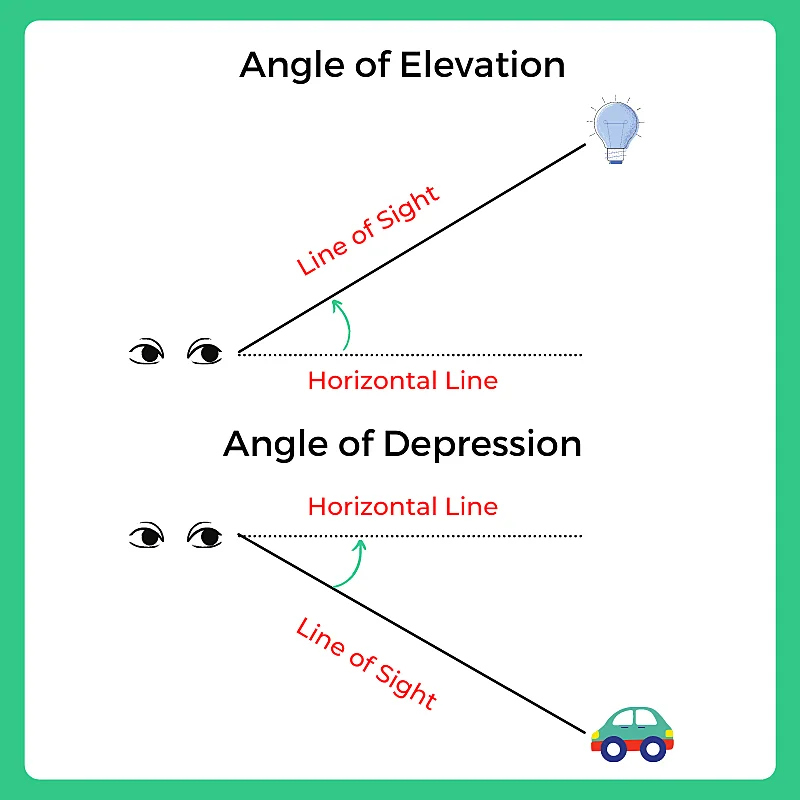### Related Banners

Get PrepInsta Prime & get Access to all 200+ courses offered by PrepInsta in One Subscription

## Also Check Out

### Circular Permutation Questions and Answers

1. A man is standing in his balcony, which is on the third floor of the building and is at the height of 10m. His angle of elevation at the top of the opposite building is 600, and the angle of depression of the base is 300. Determine the height of the building opposite to him?40 mtrs

40 mtrs

76.67%

50 mtrs

50 mtrs

6.67%

70 mtrs

70 mtrs

6.67%

100 mtrs

100 mtrs

10%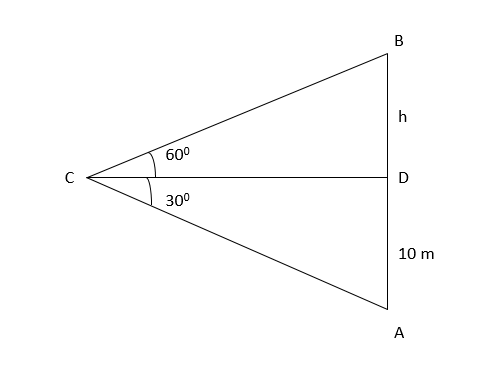Let AB the building opposite to the man.

Let us assume he is standing at point C

Therefore, we can say that  ∠BCD = 600

∠ACD = 300

Let BD = h

In the given  ADC, tan 300 = 10/CD

• 1/√3= 10/CD
• CD = 10 √3

Also, in the given  ΔDBC, we can say that

Tan 600 = h/ CD

• √3= h/ 10 √3
• H= 30 mtrs

Therefore, the height of the opposite building is

AB = BD + DA

AB = 10 + 30

AB = 40 mtrs2. A boy is standing on a riverbank. His angle of elevation from the treetop, which is on the opposite bank is 450. When he walks 20 meters away from his place, his angle of elevation becomes 300. What is the height of the tree?7 m

7 m

6.9%

5(√3 + 2)m

5(√3 + 2)m

6.9%

10(√3 + 1)m

10(√3 + 1)m

75.86%

None of the above

None of the above

10.34%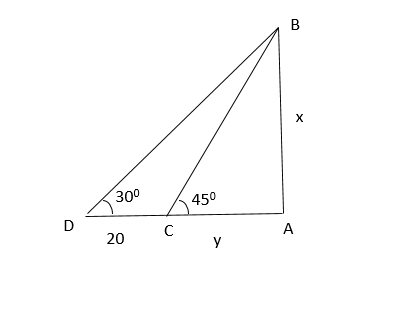Let BA = x (height of the tree)

AC = y (river)

Let the angle of elevation at point C = 450

Angle of elevation at point D = 300

CD = 20mts

Also, in the above  ΔACB,

tan 450 = x/y

• 1 = x/y
• X = y

Also, in the given above  ΔADB,

1/ √3 = x / (20 +y)

1/ √3 = x / (20 +x) (because of 1)

20 + x = √3 x

(  √3– 1) x = 20

X = 20 / (  √3– 1)

X = 10(√3 + 1) m3. Raj is standing on the top of a building which is 60 meters high. The angle of elevation and depression to the top and the foot of another building are α and β. What is the height of the second building?cot β tan α

cot β tan α

12%

60 (1 + cot β tan α)

60 (1 + cot β tan α)

64%

60 (1 + tan α)

60 (1 + tan α)

16%

None of the above

None of the above

8%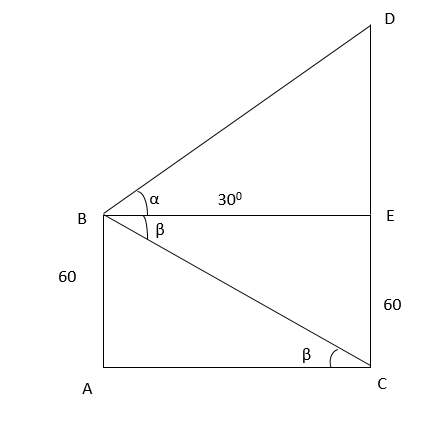In the above figure,

ΔBAC tan β = 60/ AC

BE = AC

60/ tan β = 60 cot β

Also, in the above  ΔBED

tan α = DE/ BE

tan α= DE/ 60 cot β

DE= 60 cot β tan α

Therefore, the height of the building = CD = CE + ED

= 60 + 60 cot β tan α

= 60 (1 + cot β tan α)4. From the top of a building, which is 75m high, the angles of depression of the top and the bottom of a pole which is present on the same plane as the building are 300 and 450, What is the pole’s height?75m

75m

14.81%

28.3m

28.3m

22.22%

32m

32m

18.52%

31.7m

31.7m

44.44%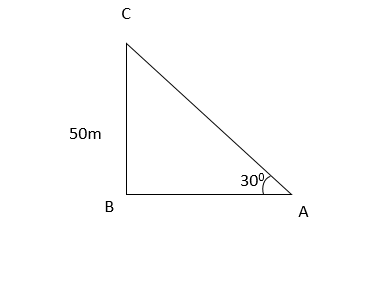The tower of height 50m.

We have to determine the distance AB

In the given  ABC, tan 300 = BC/AB

• 1/ √3 = 50/AB
• AB = 50√35. A tower is 50m high. The elevation angle to the top of the tower from the point on A on the ground is 300. Calculate the distance from the bottom of the tower and point A.50√3

50√3

82.61%

20√3

20√3

4.35%

26

26

4.35%

50√4

50√4

8.7%The tower of height 50m.

We have to determine the distance AB

In the given  ABC, tan 300 = BC/AB

• 1/ √3 = 50/AB
• AB = 50√36. A girl is standing on the ground at a point P. If she looks at the topmost point of the building, the angle of elevation is 600. If the point P is 32 m from the building, what is its height?30√5

30√5

8%

20√2

20√2

8%

32√3

32√3

80%

90√2

90√2

4%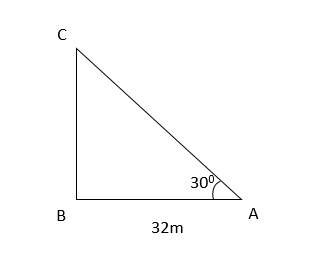Let the building be CB, and the girl is standing at A.

AB = 32 m

In the above triangle ABC, tan 300= BC/AB

• √3 = BC/AB
• BC = 32√3

Therefore, the height of the building is 32√3.7. A lighthouse is 80m high, a person observes two ships. The angles of elevation of both these ships as observed from the lighthouse are 450 and 300, What is the distance between the two ships?40.36m

40.36m

9.52%

58.56m

58.56m

52.38%

89.36m

89.36m

23.81%

None of the above

None of the above

14.29%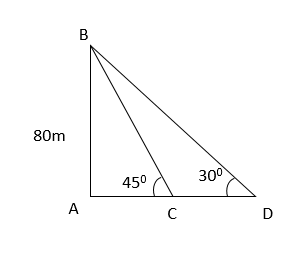We can say that BA is the height of the lighthouse and the ships are present at points C and D.

Let CD = x

tan 450 = Perpendicular/ Hypotenuse

1 = 80/AC

AC = 80m

1/√3 = 80/ (80+x)

(80+x) = 80√3

Therefore, x = 58.56m8. A building which is 20 meters high, a man is flying a kite. He is using a thread that is 75m long to keep the kite in the air. If the thread is making an angle of 450 with the horizontal, then calculate the height of the kite from the ground.70.36m

70.36m

25%

78.3m

78.3m

20.83%

56.3m

56.3m

8.33%

63.3m

63.3m

45.83%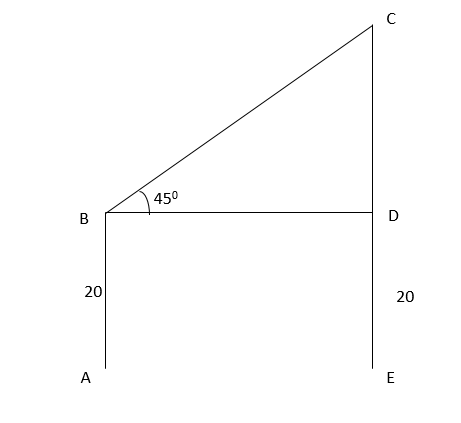Let AB be the height of the building 20m.

Let the string be BC

In triangle BDC, the value of sin 450 = CD/BC

Or,

1/√2 = CD/BC

1/√2 = CD/ 75

CD = 43.3m

Therefore, the height of the kite is 20 + 43.3 = 63.3mtr9. A ground has two poles of height 30mtrs and 10mtrs on the ground. A wire connects both these poles of length 40mtrs. What is the angle made by the wire with the horizontal?80 degree

80 degree

12.5%

30 degree

30 degree

70.83%

45 degree

45 degree

12.5%

90 degree

90 degree

4.17%

Let the two poles be AB and CD.

Let the angle made by the wire and the horizontal axis be φ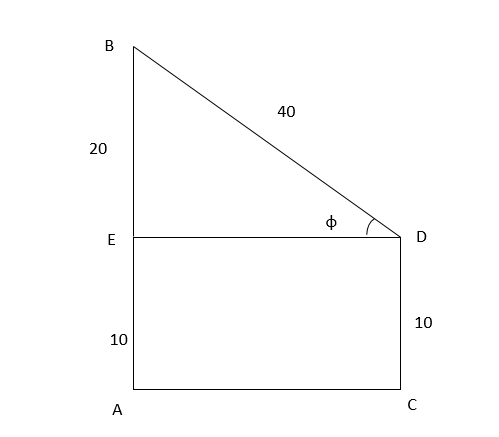In the above triangle DEB,

Sin φ = BE/BD

= 20/40

= ½

Or, we can say

Sin φ = 30010. The angles of depression of the top and bottom of a tree, as observed from the top of a building, which is 90mtrs high are 300 and 600. What is the height of the tree?60m

60m

75%

20m

20m

16.67%

10m

10m

4.17%

50m

50m

4.17%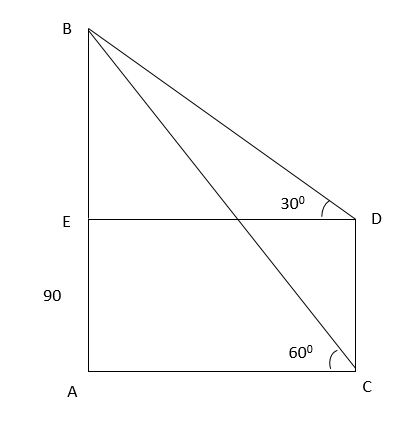Let the tower be AB of height 90mtr

Let the tree be= CD

In the above triangle ABC, tan 600 = AB/AC

AC = 30√3

Hence, DE = AC = 30√3

In the given triangle DEB,

Tan 300 = BE/DE

1/√3 = BE/ 30√3

BE = 30m

Hence, CD = AE

= 90-30= 60m×